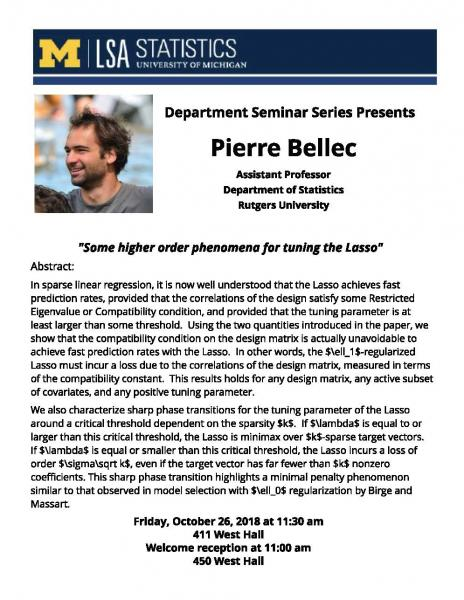Skip to Content

### Search: {{$root.lsaSearchQuery.q}}, Page {{$root.page}}

{{item.title}}
 {{item.snippet}}

# Statistics Department Seminar Series: Pierre Bellec, Assistant Professor of statistics, Rugers University

Some higher order phenomena for tuning the LassoFriday, October 26, 2018
11:30 AM-1:00 PM
411 West Hall Map
In sparse linear regression, it is now well understood that the Lasso achieves fast prediction rates, provided that the correlations of the design satisfy some Restricted Eigenvalue or Compatibility condition, and provided that the tuning parameter is at least larger than some threshold. Using the two quantities introduced in the paper, we show that the compatibility condition on the design matrix is actually unavoidable to achieve fast prediction rates with the Lasso. In other words, the $\ell_1$-regularized Lasso must incur a loss due to the correlations of the design matrix, measured in terms of the compatibility constant. This results holds for any design matrix, any active subset of covariates, and any positive tuning parameter.
We also characterize sharp phase transitions for the tuning parameter of the Lasso around a critical threshold dependent on the sparsity $k$. If $\lambda$ is equal to or larger than this critical threshold, the Lasso is minimax over $k$-sparse target vectors. If $\lambda$ is equal or smaller than this critical threshold, the Lasso incurs a loss of order $\sigma\sqrt k$, even if the target vector has far fewer than $k$ nonzero coefficients. This sharp phase transition highlights a minimal penalty phenomenon similar to that observed in model selection with $\ell_0$ regularization by Birge and Massart.
Building: West Hall Workshop / Seminar seminar Happening @ Michigan from Department of Statistics, Department of Statistics Seminar Series, Department of Statistics Graduate Seminar Series